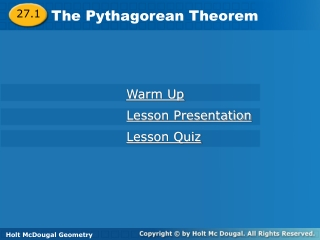# The Pythagorean Theorem - PowerPoint PPT PresentationDownload PresentationThe Pythagorean Theorem

Presentation Description
Download Presentation## The Pythagorean Theorem

- - - - - - - - - - - - - - - - - - - - - - - - - - - E N D - - - - - - - - - - - - - - - - - - - - - - - - - - -
##### Presentation Transcript

1. 27.1 The Pythagorean Theorem Warm Up Lesson Presentation Lesson Quiz Holt McDougal Geometry Holt Geometry

2. 27.1 Review Classify each triangle by its angle measures. 1.2. 3. Simplify 4. If a = 6, b = 7, and c = 12, find a2 + b2 and find c2. Which value is greater? acute right 12 85; 144; c2

3. 27.1 Objectives Use the Pythagorean Theorem and its converse to solve problems. Use Pythagorean inequalities to classify triangles.

4. 27.1 Vocabulary Pythagorean triple

5. 27.1 The Pythagorean Theorem is probably the most famous mathematical relationship. As you learned in Lesson 1-6, it states that in a right triangle, the sum of the squares of the lengths of the legs equals the square of the length of the hypotenuse. a2 + b2 = c2

6. 27.1 Example 1 Find the value of x. Give your answer in simplest radical form. a2 + b2 = c2 Pythagorean Theorem 22 + 62 = x2 Substitute 2 for a, 6 for b, and x for c. 40 = x2 Simplify. Find the positive square root. Simplify the radical.

7. 27.1 Example 2 What if...? According to the recommended safety ratio of 4:1, how high will a 30-foot ladder reach when placed against a wall? Round to the nearest inch. Let x be the distance in feet from the foot of the ladder to the base of the wall. Then 4x is the distance in feet from the top of the ladder to the base of the wall.

8. 27.1 Example 2 Continued Pythagorean Theorem a2 + b2 = c2 Substitute 4x for a, x for b, and 30 for c. (4x)2 + x2 = 302 Multiply and combine like terms. 17x2 = 900 Since 4x is the distance in feet from the top of the ladder to the base of the wall, 4(7.28)  29 ft 1 in.

9. 27.1 A set of three nonzero whole numbers a, b, and c such that a2 + b2 = c2 is called a Pythagorean triple.

10. The side lengths do not form a Pythagorean triple because is not a whole number. 27.1 Example 3 Find the missing side length. Tell if the side lengths form a Pythagorean triple. Explain. a2 + b2 = c2 Pythagorean Theorem 42 + b2 = 122 Substitute 4 for a and 12 for c. b2 = 128 Multiply and subtract 16 from both sides. Find the positive square root.

11. 27.1 The converse of the Pythagorean Theorem gives you a way to tell if a triangle is a right triangle when you know the side lengths.

12. B c a A C b 27.1 You can also use side lengths to classify a triangle as acute or obtuse.

13. Remember! By the Triangle Inequality Theorem, the sum of any two side lengths of a triangle is greater than the third side length. 27.1

14. 27.1 Example 4 Tell if the measures can be the side lengths of a triangle. If so, classify the triangle as acute, obtuse, or right. 5, 7, 10 Step 1 Determine if the measures form a triangle. By the Triangle Inequality Theorem, 5, 7, and 10 can be the side lengths of a triangle.

15. ? c2 = a2 + b2 ? 102 = 52 + 72 ? 100 = 25 + 49 27.1 Example 4 Step 2 Classify the triangle. Compare c2 to a2 + b2. Substitute the longest side for c. Multiply. 100 > 74 Add and compare. Since c2 > a2 + b2, the triangle is obtuse.

16. Since 5 + 8 = 13 and 13 > 17, these cannot be the side lengths of a triangle. 27.1 Example 5 Tell if the measures can be the side lengths of a triangle. If so, classify the triangle as acute, obtuse, or right. 5, 8, 17 Step 1 Determine if the measures form a triangle.

17. 27.1 Example 6 Tell if the measures can be the side lengths of a triangle. If so, classify the triangle as acute, obtuse, or right. 3.8, 4.1, 5.2 Step 1 Determine if the measures form a triangle. By the Triangle Inequality Theorem, 3.8, 4.1, and 5.2 can be the side lengths of a triangle.

18. ? c2 = a2 + b2 ? 5.22 = 3.82 + 4.12 ? 27.04 = 14.44 + 16.81 27.1 Example 6 Step 2 Classify the triangle. Compare c2 to a2 + b2. Substitute the longest side for c. Multiply. 27.04 < 31.25 Add and compare. Since c2 < a2 + b2, the triangle is acute.

19. 27.1 Lesson Quiz: Part I 1. Find the value of x. 2. An entertainment center is 52 in. wide and 40 in. high. Will a TV with a 60 in. diagonal fit in it? Explain. 12

20. 27.1 Lesson Quiz: Part II 3. Find the missing side length. Tell if the side lengths form a Pythagorean triple. Explain. 4. Tell if the measures 7, 11, and 15 can be the side lengths of a triangle. If so, classify the triangle as acute, obtuse, or right. 13; yes; the side lengths are nonzero whole numbers that satisfy Pythagorean’s Theorem. yes; obtuse